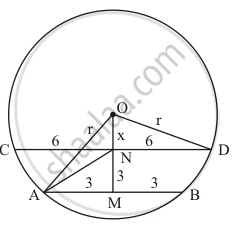Advertisement Remove all ads

# Ab and Cd Are Two Parallel Chords of a Circle with Centre O Such that Ab = 6 Cm and Cd = 12 Cm. the Chords Are on Same Side of the Centre and Distance Between Them is 3 Cm. the Radius of the Circle is - Mathematics

MCQ

AB and CD are two parallel chords of a circle with centre O such that AB = 6 cm and CD= 12 cm. The chords are on the same side of the centre and the distance between them is 3 cm. The radius of the circle is

#### Options

• 6 cm

• $5\sqrt{2} cm$

•  7 cm

• $3\sqrt{5} cm$

Advertisement Remove all ads

#### Solution

$3\sqrt{5}cm$
Let distance between the centre and the chord CD be x cm and the radius of the circle is r cm.
We have to find the radius of the following circle:In triangle OND,

x^2 + 36 = r^2 …… (1)

Now, in triangle AOM,

r^2 = 9 + (x +3)^2 …… (2)

From (1) and (2), we have,

r^2 = 9 + (sqrtr^2 - 36 + 3)^2

⇒ r^2 = 9 + r^2 - 36 + 9 + 6 sqrt(r^2 - 36 )

⇒ 3 = sqrt(r^2 - 36)

⇒9 = r^2 - 36

⇒r^2 = 45 ⇒ r =3sqrt(5)

Is there an error in this question or solution?
Advertisement Remove all ads

#### APPEARS IN

RD Sharma Mathematics for Class 9
Chapter 15 Circles
Q 28 | Page 112
Advertisement Remove all ads

#### Video TutorialsVIEW ALL 

Advertisement Remove all ads
Share
Notifications

View all notifications

Forgot password?
Course